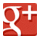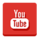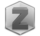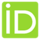You are in:Home/Theses

### Ass. Lect. Mohamed Shehata Saleh Khalil :: Theses :

 Title Design of Wind Turbine Drive Train Based on Dynamic Lagrange Multipliers Type MSc Supervisors Prof. Dr. Ahmed M. El-Assal, Assoc. Prof. Ahmed A. El-Betar Year 2015 Abstract This thesis is aimed to develop a mathematical model used as design tool in order to increase the efficiency of Wind Turbines Drive Train (WTDT) using spatial multibody formulation with Euler parameter coordinates. The multibody model correctly describes the motion and inertial characteristics of simple/complex drive train. Using lagrange multipliers associated with constraints of multibody systems can calculate the reaction forces into drive train components, which enables stress analysis and design process can be establish. In recent years, mathematical models for multibody dynamic systems have become an important design and analysis tool. Multibody dynamic systems are presented by series of rigid and/or flexible links containing a wide variety of joint types. The mathematical models are defined by differential algebraic equations DAE which can simulate large rotation systems. Several general-purpose computer programs exist, which allow engineers to model complex mechanical systems and to evaluate the dynamic characteristics of potential designs before building of prototypes. This reduces cost and lead time in designs. These general-purpose simulation packages have several drawbacks. They need to be operated by experienced engineers and are numerically inefficient. As a result, extensive research efforts have been directed to developing more efficient and user-friendly methods for modeling multibody dynamic systems. In this work, Multibody computational code is developed using MATLAB. Moreover, symbolic derivation has been carried out to derive explicit equation of motion of wind turbines drive train. These equations are then available for numerical integration, consequently calculate the dynamic forces to help in the design process of such systems, The results contain angular velocities and reaction forces of drive train. A rigid multibody dynamic model of a horizontal wind turbine drive train used in this work is composed of rotor, gearbox, and generator. Gearbox is the most important element in drive train which is composed of a planetary and a conventional parallel axis gear. This subsystem is formulated to study the system dynamic behavior as an integrated complete system. Multibody modeling of gear sets allowing the dynamic analysis to establish design by computing the forces acting on gear body and gear teeth. MATLAB model results were compared using MSC ADAMS software by using actual WTDT case study parameter with grate similarity. Keywords Multibody System Dynamics, University Benha University Country Egypt Full Paper download paper